# Error message in PSpice

Louis Aguilar

## Homework Statement

Run a transient simulation and plot the three outputs, Vo(t), on the same plot. Use the following settings:
1. Set the Run Time (or Stop Time) so that you plot 3 cycles of Vo(t).
2. Set the Maximum Step Size so that you get at least 100 points per period for Vo(t).
3. Set the Y axis limits to 0 volts and 10 volts. Do not auto scale.
The model that you will use for the MOSFET is:
Id = (kp/2)(Vgs – Vto)2 (1 + lambda*Vds)
Kp = 0.01
Vto = 1
Lambda = 0.05

Given Circuit Design: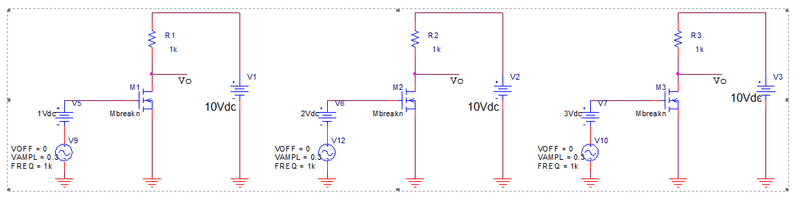## Homework Equations

I have attempted to do it in PSpice but I'm getting error code saying missing values, what am I doing wrong?

## The Attempt at a Solution

My Design: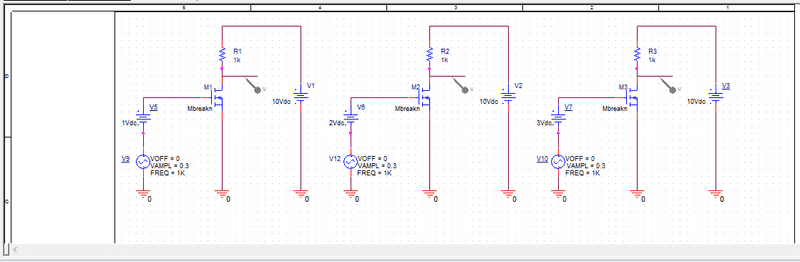My Simulation Profile: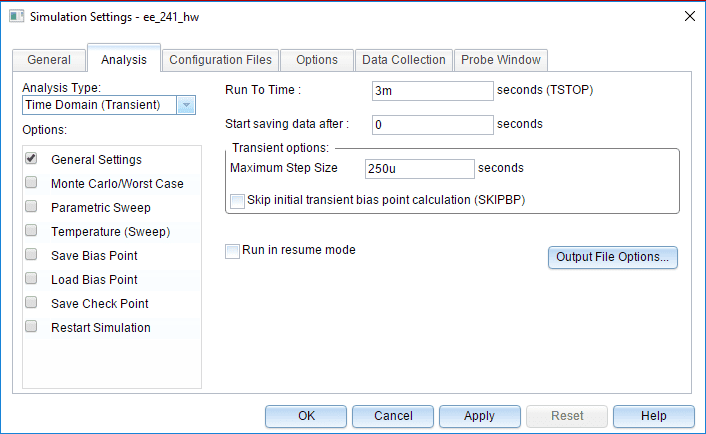Mosfet PSpice Model: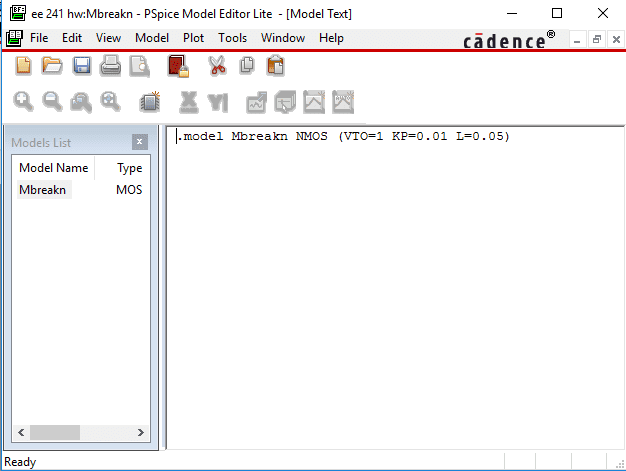Error Code: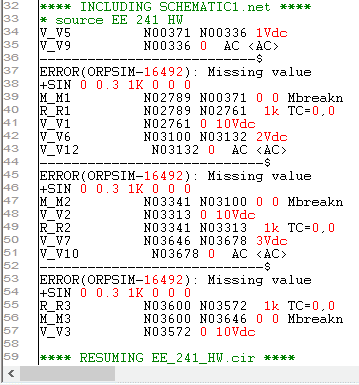Mentor

## Homework Statement

Run a transient simulation and plot the three outputs, Vo(t), on the same plot. Use the following settings:
1. Set the Run Time (or Stop Time) so that you plot 3 cycles of Vo(t).
2. Set the Maximum Step Size so that you get at least 100 points per period for Vo(t).
3. Set the Y axis limits to 0 volts and 10 volts. Do not auto scale.
The model that you will use for the MOSFET is:
Id = (kp/2)(Vgs – Vto)2 (1 + lambda*Vds)
Kp = 0.01
Vto = 1
Lambda = 0.05

Given Circuit Design:
View attachment 196978

## Homework Equations

I have attempted to do it in PSpice but I'm getting error code saying missing values, what am I doing wrong?

## The Attempt at a Solution

My Design:
View attachment 196979
My Simulation Profile:
View attachment 196980
Mosfet PSpice Model:
View attachment 196982
Error Code:
View attachment 196981
Welcome to the PF.

What does the manual say about that error 16492? Can you simplify the simulation down to just one circuit for now, and see if you can figure out what's missing?